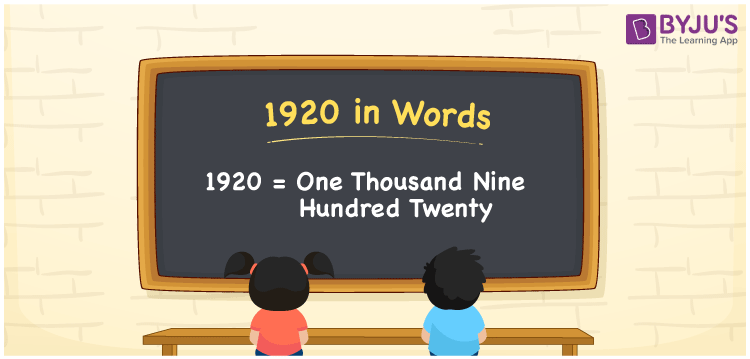# 1920 in Words

1920 in words is written as One thousand nine hundred twenty. In both the International System of Numerals and the Indian System of Numerals, 1920 is written as One thousand nine hundred twenty.

We often use the number 1920 as “1920 plants were planted”. 1920 describes a quantity, “how much of something” or “how many of something”. Hence, the number 1920 is a Cardinal Number.

 1920 in Words One thousand nine hundred twenty One thousand nine hundred twenty in Number 1920

## 1920 in English Words

1920 in English words is read as “One thousand nine hundred twenty.”## How to Write 1920 in Words?

To write 1920 in words, we shall use the place value chart. In the place value chart, put 1 in the thousands, 9 in the hundreds, 2 in the tens and 0 in the ones, respectively. Let us make a place value chart to write the number 1920 in words.

 Thousands Hundreds Tens Ones 1 9 2 0

Thus, we can write the expanded form as

1 × Thousand + 9 × Hundred + 2 × Ten + 0 × One

= 1 × 1000 + 9 × 100 + 2 × 10 + 0 × 1

= 1000 + 900 + 20 + 0

= 1920

= One thousand nine hundred twenty.

1920 is the natural number that is succeeded by 1919 and preceded by 1921.

1920 in words – One thousand nine hundred twenty

• Is 1920 an odd number? – No
• Is 1920 an even number? – Yes
• Is 1920 a perfect square number? – No
• Is 1920 a perfect cube number? – No
• Is 1920 a prime number? – No
• Is 1920 a composite number? – Yes

## Frequently Asked Questions on 1920 in Words

Q1

### How to write 1920 in words?

1920 in words is written as One thousand nine hundred twenty.
Q2

### How to write 1920 in words in the International and Indian System of Numerals?

In both, the system of numerals, 1920 in words, is written as One thousand nine hundred twenty.
Q3

### How to write 1920 in a place value chart?

In the place value chart, write 1 in the thousands, 9 in the hundreds, 2 in the tens and 0 in the ones, respectively.